﻿ 基于近似贝叶斯方法的AR(p)模型参数估计问题研究

# 基于近似贝叶斯方法的AR(p)模型参数估计问题研究Research on Parameter Estimation of AR(p) Model Based on Approximate Bayesian Computation

Abstract: In this paper, approximate Bayesian computation and least square method are used to estimate the parameters of AR(p) model. The simulation AR(2) model data are generated by RStudio software, and the accuracy of parameter estimate is compared between the two methods. Finally, the effectiveness of the two parameter estimation methods is verified by examples.

1. 引言

2. 模型介绍

2.1. AR(p)模型

$f\left(\lambda \right)=1-\underset{i=1}{\overset{p}{\sum }}{\alpha }_{i}{\lambda }^{i}=0$ 的根都落在单位圆外，则称 $\left\{{Y}_{t}\right\}$ 为平稳序列过程。

2.2. AR(p)模型参数的最小二乘估计

$\left(\begin{array}{cccc}1& {\rho }_{1}& \cdots & {\rho }_{p-1}\\ {\rho }_{1}& 1& \cdots & {\rho }_{p-2}\\ ⋮& ⋮& \ddots & ⋮\\ {\rho }_{p-1}& {\rho }_{p-2}& \cdots & 1\end{array}\right)\left(\begin{array}{c}{\alpha }_{1}\\ {\alpha }_{2}\\ ⋮\\ {\alpha }_{p}\end{array}\right)=\left(\begin{array}{c}{\rho }_{1}\\ {\rho }_{2}\\ ⋮\\ {\rho }_{p}\end{array}\right)$

$\left(\begin{array}{c}\stackrel{^}{{\alpha }_{1}}\\ \stackrel{^}{{\alpha }_{2}}\\ ⋮\\ \stackrel{^}{{\alpha }_{p}}\end{array}\right)={\left(\begin{array}{cccc}1& {\rho }_{1}& \cdots & {\rho }_{p-1}\\ {\rho }_{1}& 1& \cdots & {\rho }_{p-2}\\ ⋮& ⋮& \ddots & ⋮\\ {\rho }_{p-1}& {\rho }_{p-2}& \cdots & 1\end{array}\right)}^{-1}\left(\begin{array}{c}{\rho }_{1}\\ {\rho }_{2}\\ ⋮\\ {\rho }_{p}\end{array}\right)$

$\stackrel{^}{{\sigma }^{2}}=\frac{1}{n-p}\underset{t=p+1}{\overset{m}{\sum }}{\left({Y}_{t}-\stackrel{^}{{\alpha }_{1}}{Y}_{t-1}-\cdots -\stackrel{^}{{\alpha }_{p}}{Y}_{t-p}\right)}^{2}$

2.3. AR(p)模型参数的近似贝叶斯估计

2.3.1. 近似贝叶斯基本思想

2.3.2. ABC拒绝算法

ABC方法的最基本形式是ABC拒绝算法。步骤如下：

1) 从先验分布 $p\left(\alpha \right)$ 中生成一个 ${\alpha }^{*}$，作为一个样本；

2) 由 ${\alpha }^{*}$ 根据模型Q生成模拟数据集 ${Y}^{*}$

3) 计算 $d\left(Y,{Y}^{*}\right)$，若 $d\left(Y,{Y}^{*}\right)\le \epsilon$，则接受 ${\alpha }^{*}$，令 ${\alpha }_{1}={\alpha }^{*}$ 并记录下来；反之则拒绝 ${\alpha }^{*}$

4) 重复步骤1)-3)，直到有n个样本被接受；

5) 计算参数 $\alpha$ 的估计值 $\stackrel{^}{\alpha }$$\stackrel{^}{\alpha }=\frac{1}{n}\underset{i=1}{\overset{n}{\sum }}{\alpha }_{i}$

2.3.3. AR(p)模型参数的ABC估计算法

1) 计算 ${y}_{1},{y}_{2},\cdots ,{y}_{m}$ 的自相关系数 ${\rho }_{1},{\rho }_{2},\cdots ,{\rho }_{p}$

2) 根据先验分布 $p\left(\alpha \right)$ 生成一组 ${\alpha }_{1},{\alpha }_{2},\cdots ,{\alpha }_{p}$

3) 计算 $\alpha$ 特征方程的根 ${\lambda }_{i},i=1,\cdots ,p$。若 $\forall |{\lambda }_{i}|>1$，则到步骤(4)；否则退回步骤(2)。

4) 根据 ${\alpha }_{1},{\alpha }_{2},\cdots ,{\alpha }_{p}$$\sigma ={\sigma }_{0}$ 生成AR(p)模型数据 ${{y}^{\prime }}_{1},{{y}^{\prime }}_{2},\cdots ,{{y}^{\prime }}_{m}$

5) 计算数据 ${{y}^{\prime }}_{1},{{y}^{\prime }}_{2},\cdots ,{{y}^{\prime }}_{m}$ 的自相关系数 ${{\rho }^{\prime }}_{1},{{\rho }^{\prime }}_{2},\cdots ,{{\rho }^{\prime }}_{p}$

6) 计算 $d\left(\rho ,{\rho }^{\prime }\right)=\sqrt{\underset{i=1}{\overset{p}{\sum }}{\left({\rho }_{i}-{{\rho }^{\prime }}_{i}\right)}^{2}}$。若 $d\left(\rho ,{\rho }^{\prime }\right)\le \epsilon$，则接受 ${\alpha }_{1},{\alpha }_{2},\cdots ,{\alpha }_{p}$ 作为一组样本，令 ${\alpha }_{11}={\alpha }_{1},\cdots ,{\alpha }_{p1}={\alpha }_{p}$ 并记录下来；反之则拒绝 ${\alpha }_{1},{\alpha }_{2},\cdots ,{\alpha }_{p}$

7) 重复步骤2)-6)，直到有n组样本被接受；

8) 计算参数 $\alpha$ 的估计值 $\stackrel{^}{\alpha }$$\stackrel{^}{{\alpha }_{i}}=\frac{1}{n}\underset{j=1}{\overset{n}{\sum }}{\alpha }_{ij},i=1,\cdots ,p$

1) 计算 ${y}_{1},{y}_{2},\cdots ,{y}_{m}$ 的自相关系数 ${\rho }_{1},{\rho }_{2},\cdots ,{\rho }_{p}$

2) 根据先验分布 $p\left(\sigma \right)$ 生成一个 ${\sigma }^{*}$

3) 根据 $\stackrel{^}{{\alpha }_{1}},\stackrel{^}{{\alpha }_{2}},\cdots ,\stackrel{^}{{\alpha }_{p}}$$\sigma ={\sigma }^{*}$ 生成AR(p)模型数据 ${{y}^{\prime }}_{1},{{y}^{\prime }}_{2},\cdots ,{{y}^{\prime }}_{m}$

4) 计算数据 ${{y}^{\prime }}_{1},{{y}^{\prime }}_{2},\cdots ,{{y}^{\prime }}_{m}$ 的自相关系数 ${{\rho }^{\prime }}_{1},{{\rho }^{\prime }}_{2},\cdots ,{{\rho }^{\prime }}_{p}$

5) 计算 $d\left(\rho ,{\rho }^{\prime }\right)=\sqrt{\underset{i=1}{\overset{p}{\sum }}{\left({\rho }_{i}-{{\rho }^{\prime }}_{i}\right)}^{2}}$。若 $d\left(\rho ,{\rho }^{\prime }\right)\le \epsilon$，则接受 ${\sigma }^{*}$，令 ${\sigma }_{1}={\sigma }^{*}$ 并记录下来；反之则拒绝 ${\sigma }^{*}$

6) 重复步骤(2)-(5)，直到有n个 ${\sigma }^{*}$ 被接受；

7) 计算参数 $\sigma$ 的估计值 $\stackrel{^}{\sigma }$$\stackrel{^}{\sigma }=\frac{1}{n}\underset{i=1}{\overset{n}{\sum }}{\sigma }_{i}$

3. 模拟验证

${Y}_{t}=0.3{Y}_{t-1}-0.4{Y}_{t-2}+{\epsilon }_{t},t=1,\cdots ,m$

RStudio软件中arima函数便是使用最小二乘法来估计参数的，因此我们直接调用arima函数去估计AR(2)模型的参数。Figure 1. Parameter alpha frequency distribution histogram and posterior distribution density function curve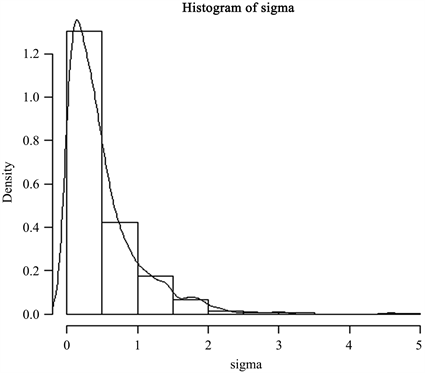Figure 2. Parameter sigma frequency distribution histogram and posterior distribution density function curveTable 1. Parameter estimate of AR(2) model

4. 实例分析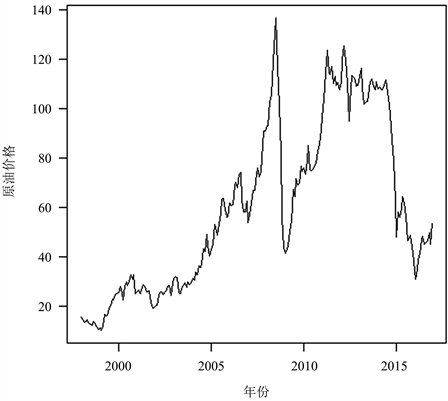Figure 3. Crude oil futures closing price series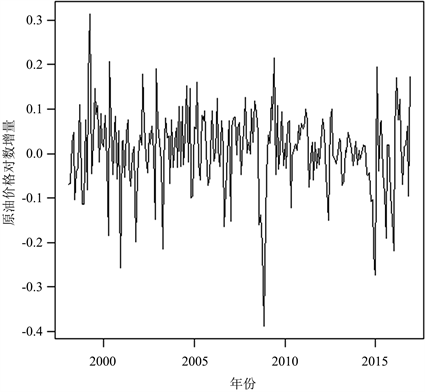Figure 4. Crude oil futures closing price’s logarithm increment series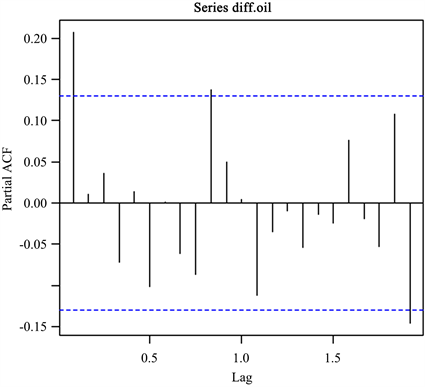Figure 5. PACF diagram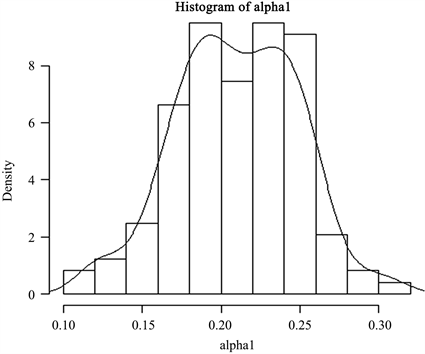Figure 6. Parameter alpha1 frequency distribution histogram and posterior distribution density function curve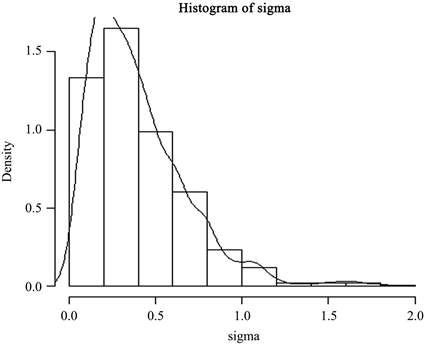Figure 7. Parameter sigma frequency distribution histogram and posterior distribution density function curve

5. 总结

 王玉丽, 包为民, 沈丹丹, 等. AR模型参数的抗差递推估计[J]. 中国农村水利水电, 2017(6): 74-77.

 王志坚. 稳健AR模型的构建及其在金融时序中的应用[J]. 统计与信息论坛, 2017, 32(5): 57-63.

 王志坚, 王斌会. 稳健AR模型的构建及比较研究[J]. 数学的实践与认识, 2019(13): 156-166.

 朱慧明, 韩玉启, 郑进城. 基于正态Gamma共轭先验分布的贝叶斯AR(p)预测模型[J]. 统计与决策, 2005(2): 10-11.

 章敏, 李再兴. AR(1)模型中参数估计的偏差分析[J]. 统计与决策, 2016(6): 26-28.

 彭毳鑫, 胡敏, 赵志文. 条件矩约束下一阶自回归模型的参数经验似然推断[J]. 统计与决策, 2018, 34(21): 33-37.

 陈阳, 夏志明, 高海菊. AR(p)模型参数估计和定阶的Lasso方法[J]. 西北大学学报: 自然科学网络版, 2011, 9(6): 483.

 谢琍, 唐甜, 王晓瑞. 线性空间自回归模型的不同惩罚函数下参数估计的比较及其实证分析[J]. 数理统计与管理, 2019(5): 823-835.

 Rubin, D.B. (1984) Bayesianly Justifiable and Relevant Frequency Calculations for the Applies Statistician. Annals of Statistics, 12, 1151-1172.
https://doi.org/10.1214/aos/1176346785

 钱瑾. 基于合成似然的近似贝叶斯方法[D]: [硕士学位论文]. 上海: 华东理工大学, 2017.

 张晨. 基于近似贝叶斯计算的参数估计和模型选择的研究[D]: [硕士学位论文]. 合肥: 合肥工业大学, 2019.

 张岚, 钱夕元. 基于近似贝叶斯计算方法的排队模型参数估计[J]. 上海理工大学学报, 2020, 42(2): 108-114.

 黄鹂. 近似贝叶斯方法及其应用研究[D]: [硕士学位论文]. 苏州: 苏州大学, 2018.

 周双酉. 基于HMC的合成似然近似贝叶斯及其应用[D]: [硕士学位论文]. 上海: 华东理工大学, 2019.

 王振龙, 胡永宏. 应用时间序列分析[M]. 北京: 科学出版社, 2007: 98-99.

 陈杨林, 刘业. AR(p)模型参数估计方法比较和实证分析[J]. 南昌大学学报(理科版), 2014(38): 127.

 Pritchard, J.K., Seielstad, M.T., Perez-Lezaun, A., et al. (1999) Population Growth of Human Y Chromosomes: A Study of Y Chromosome Microsatellites. Molecular Biology & Evolution, 16, 1791-1798.
https://doi.org/10.1093/oxfordjournals.molbev.a026091

Top